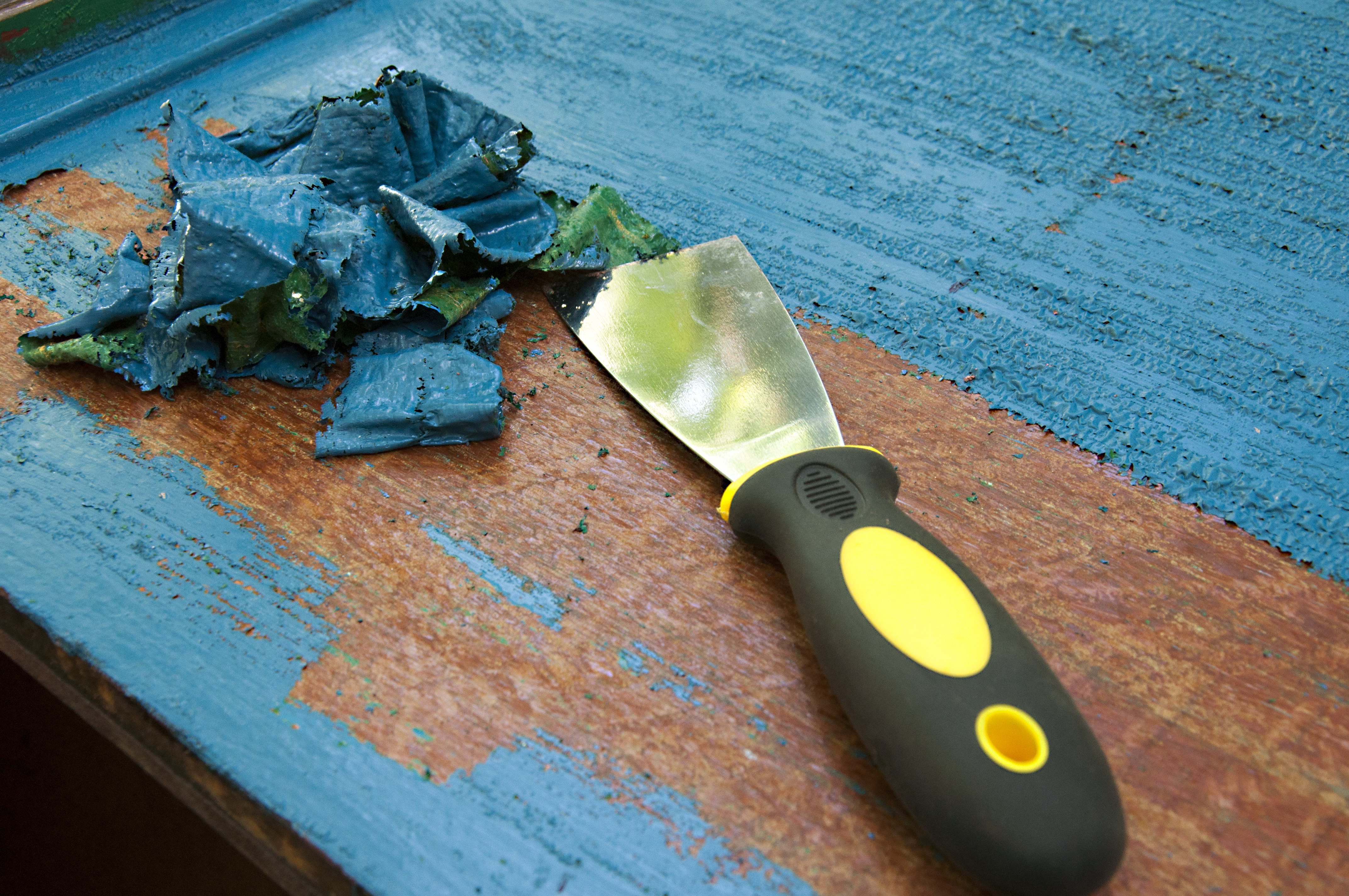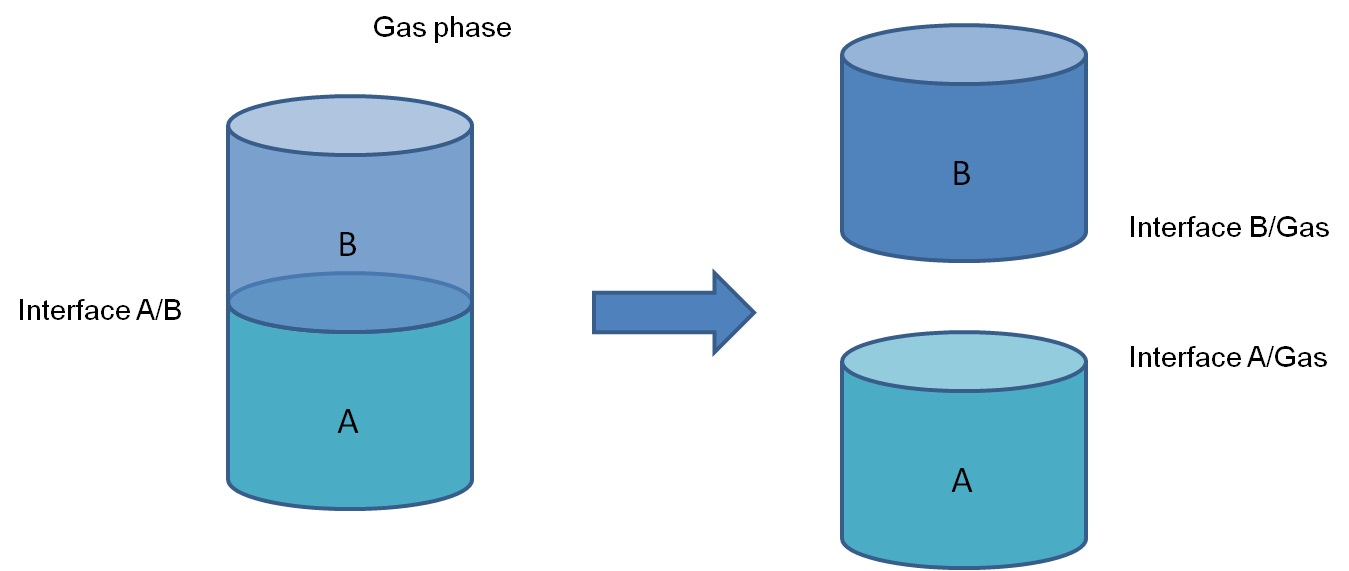Susanna Laurén Jun 23, ’20 > 2 min

# Work of adhesion - What is it and how to measure it?

## Surface Science Blog

Work of adhesion is defined as a work required to separate two phases from each other. When the two phases are in contact with each other, there is an interface between them with a certain interfacial energy, γAB. When the two phases are separated, this interface will disappear but two new are formed. These are the interface between phase A and air (γA) and the interface between phase B and air (γB). The work that is required to separate these is work of adhesion and described by Dupré as: WAB = γA + γB – γAB.

Now, if the two phases are solid and liquid, the equation can be written as

WSL = γS + γL – γSL

,where γS is the surface free energy of the solid, γL is the surface tension of a liquid and γSL is the interfacial tension between solid and the liquid.## Young - Dupré equation can be used to simplify the calculations

While the surface tension of the liquid is easy to measure, the other two are more cumbersome. To simplify the measurement of work of adhesion, the Dupré equation can be combined with the Young equation; γS = γSL + γLcosθY

which then gives Young-Dupré equation

WSL = γL(cosθ + 1).

This equation makes it possible to easily access the work of adhesion with simple contact angle measurements.

For example, work of adhesion between water drop and a glass slide would be measured by measuring the contact angle of water on the glass surface. For surface tension of water, you can use a literature value or measure it. With this information, the work of adhesion can be calculated.## Explore the blog

You have only scratched the surface.